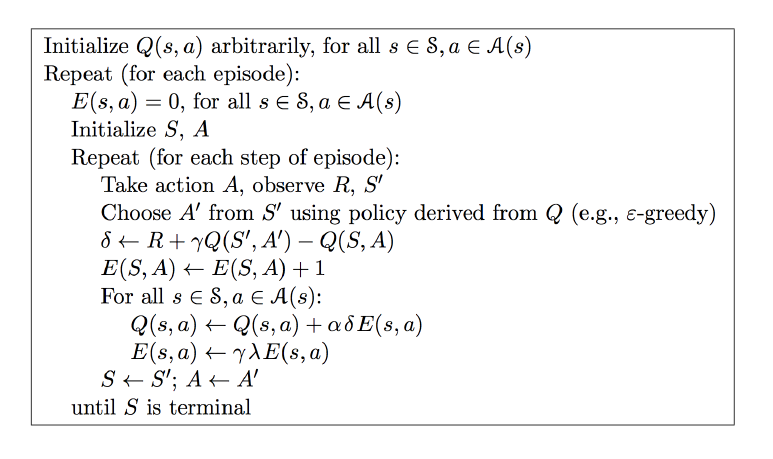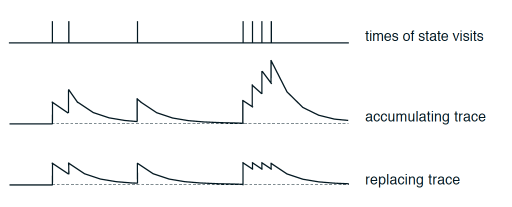# Sarsa-lambda

## 要点 ¶

Sarsa-lambda 是基于 Sarsa 方法的升级版, 他能更有效率地学习到怎么样获得好的 reward. 如果说 Sarsa 和 Qlearning 都是每次获取到 reward, 只更新获取到 reward 的前一步. 那 Sarsa-lambda 就是更新获取到 reward 的前 lambda 步. lambda 是在 [0, 1] 之间取值,

## 代码主结构 ¶

class SarsaLambdaTable:
# 初始化 (有改变)
def __init__(self, actions, learning_rate=0.01, reward_decay=0.9, e_greedy=0.9, trace_decay=0.9):

# 选行为 (与之前一样)
def choose_action(self, observation):

# 学习更新参数 (有改变)
def learn(self, s, a, r, s_):

# 检测 state 是否存在 (有改变)
def check_state_exist(self, state):## 预设值 ¶

class SarsaLambdaTable(RL): # 继承 RL class
def __init__(self, actions, learning_rate=0.01, reward_decay=0.9, e_greedy=0.9, trace_decay=0.9):
super(SarsaLambdaTable, self).__init__(actions, learning_rate, reward_decay, e_greedy)

# 后向观测算法, eligibility trace.
self.lambda_ = trace_decay
self.eligibility_trace = self.q_table.copy()    # 空的 eligibility trace 表


## 检测 state 是否存在 ¶

check_state_exist 和之前的是高度相似的. 唯一不同的地方是我们考虑了 eligibility_trace,

class SarsaLambdaTable(RL): # 继承 RL class
def __init__(self, actions, learning_rate=0.01, reward_decay=0.9, e_greedy=0.9, trace_decay=0.9):
...
def check_state_exist(self, state):
if state not in self.q_table.index:
# append new state to q table
to_be_append = pd.Series(
 * len(self.actions),
index=self.q_table.columns,
name=state,
)
self.q_table = self.q_table.append(to_be_append)

# also update eligibility trace
self.eligibility_trace = self.eligibility_trace.append(to_be_append)


## 学习 ¶

class SarsaLambdaTable(RL): # 继承 RL class
def __init__(self, actions, learning_rate=0.01, reward_decay=0.9, e_greedy=0.9, trace_decay=0.9):
...
def check_state_exist(self, state):
...
def learn(self, s, a, r, s_, a_):
# 这部分和 Sarsa 一样
self.check_state_exist(s_)
q_predict = self.q_table.ix[s, a]
if s_ != 'terminal':
q_target = r + self.gamma * self.q_table.ix[s_, a_]
else:
q_target = r
error = q_target - q_predict

# 这里开始不同:
# 对于经历过的 state-action, 我们让他+1, 证明他是得到 reward 路途中不可或缺的一环
self.eligibility_trace.ix[s, a] += 1

# Q table 更新
self.q_table += self.lr * error * self.eligibility_trace

# 随着时间衰减 eligibility trace 的值, 离获取 reward 越远的步, 他的"不可或缺性"越小
self.eligibility_trace *= self.gamma*self.lambda_


# 上面代码中的方式:
self.eligibility_trace.ix[s, a] += 1

# 更有效的方式:
self.eligibility_trace.ix[s, :] *= 0
self.eligibility_trace.ix[s, a] = 1self.eligibility_trace.ix[s, a] += 1

self.eligibility_trace.ix[s, :] *= 0; self.eligibility_trace.ix[s, a] = 1

for episode in range(100):
...

# 新回合, 清零
RL.eligibility_trace *= 0

while True: # 开始回合
...